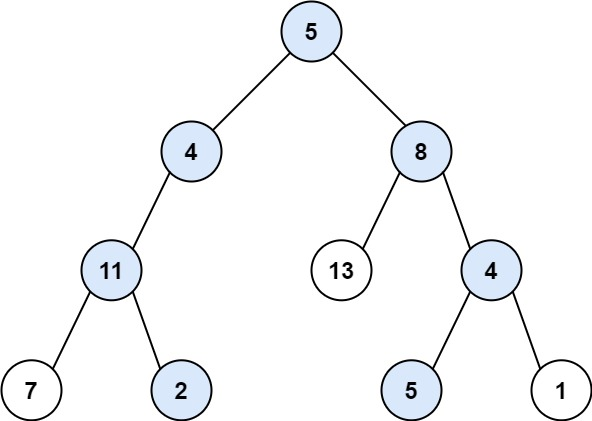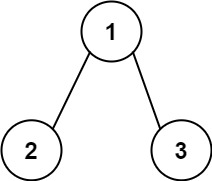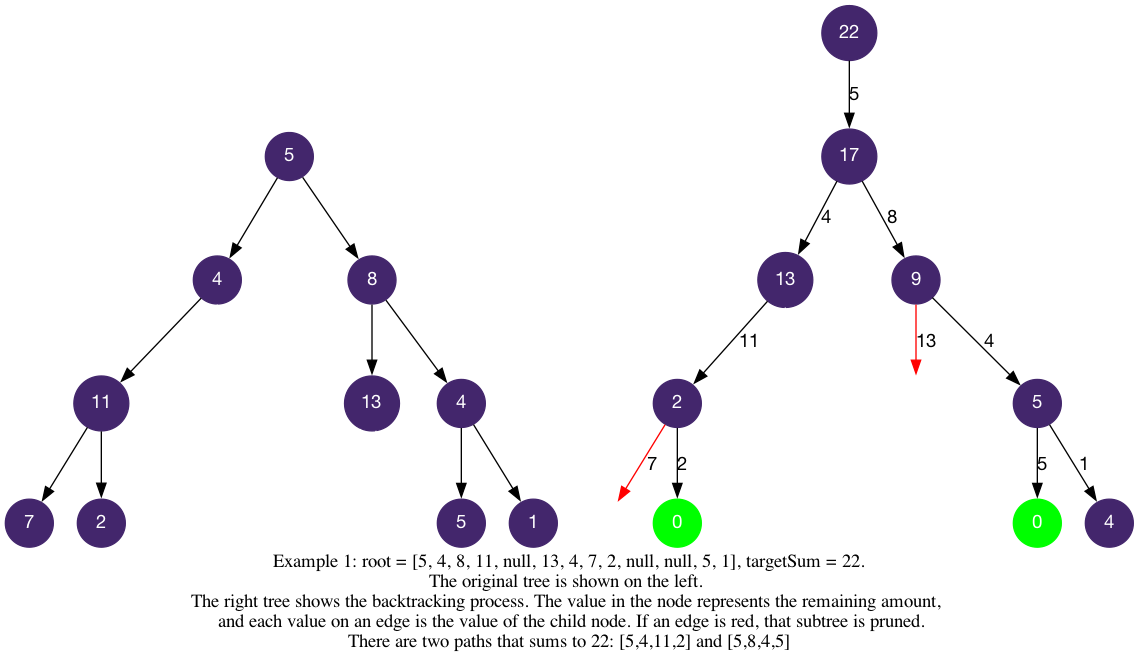# LeetCode Path Sum II Solution

Given the `root` of a binary tree and an integer `targetSum`, return all root-to-leaf paths where the sum of the node values in the path equals `targetSum`. Each path should be returned as a list of the node values, not node references.

A root-to-leaf path is a path starting from the root and ending at any leaf node. A leaf is a node with no children.

Example 1:Input: `root = [5,4,8,11,null,13,4,7,2,null,null,5,1], targetSum = 22`

Output: `[[5,4,11,2], [5,8,4,5]]`

Explanation: There are two paths whose sum equals targetSum:

5 + 4 + 11 + 2 = 22

5 + 8 + 4 + 5 = 22

Example 2:Input: `root = [1,2,3], targetSum = 5`

Output: `[]`

Example 3:

Input: `root = [1,2], targetSum = 0`

Output: `[]`

Constraints:

• The number of nodes in the tree is in the range `[0, 5000]`.
• `-1000 <= Node.val <= 1000`
• `-1000 <= targetSum <= 1000`

## Solution

We wish to fill in the template logic:

• `is_leaf`: when `node` is a leaf in the tree, and there is no remaining left.
• `get_edges`: the children of the current `node` (`node.left` and `node.right`).
• `is_valid`: an edge (node) is only invalid when it is non-empty (`None`, `null`).

In the implementation, we want to check whether `node is None` first, so that we do not try to get the field of an empty object. On the current `node`, we calculate the remaining value after adding the current value. Then we check whether the `node` is a leaf so that `path` is a root-to-leaf path and whether the `remaining` value left is 0. If these condition is satisfied, then we have found one solution. If not, we'd have to traverse further until we reach a leaf in the tree (may or may not be a solution).

The below implementation may look different than the template, but essentially one can update and revert `path` and `remaining` inside the if-else conditions to gain similarity to the template. We had also left the check of `node is None` on the outside to accommodate the use of `node.val` (and to prevent the `root` being empty).

#### Implementation

``````1def pathSum(self, root: Optional[TreeNode], targetSum: int) -> List[List[int]]:
2    def dfs(node, remaining, path):
3        if (node is None): return
4        path.append(node.val)     # update path
5        remaining -= node.val
6        if node.left is None and node.right is None and remaining == 0: # is_leaf
7            paths.append(path[:])
8        else:     # edges = [node.left, node.right]
9            dfs(node.left, remaining, path)
10            dfs(node.right, remaining, path)
11        path.pop()                # revert path
12
13    paths = []
14    dfs(root, targetSum, [])
15    return paths``````